library(rrr)

##
## Attaching package: 'rrr'

## The following object is masked from 'package:stats':
##
##     residuals


## Classical Multivariate Regression

Let $$\mathbf{X} = \left(X_1, X_2, \dots, X_r\right)^\tau$$ and $$\mathbf{Y} = \left(Y_1, Y_2, \dots, Y_s\right)^\tau$$, i.e., $$\mathbf{X}$$ is a random vector. The classical multivariate regression model is given by

$\overset{s \times 1}{\mathbf{Y}} = \overset{s \times 1}{\boldsymbol{\mu}} + \overset{s \times r}{\mathbf{C}} \; \overset{r \times 1}{\mathbf{X}} + \overset{s \times 1}{\varepsilon}$

with

$\mathrm{E}\left(\varepsilon\right) = \mathbf{0}, \quad \mathrm{cov}\left(\varepsilon\right) = \mathbf{\Sigma}_{\varepsilon \varepsilon}$

and $$\varepsilon$$ is distributed independently of $$\mathbf{X}.$$

To estimate $$\boldsymbol{\mu}$$ and $$\mathbf{C}$$ we minimize the least-squares criterion

$\mathrm{E}\left[\left(\mathbf{Y} - \boldsymbol{\mu} - \mathbf{C} \mathbf{X}\right)\left(\mathbf{Y} - \boldsymbol{\mu} - \mathbf{C}\mathbf{X}\right)^\tau\right],$

with expecation taken over the joint distribution of $$\left(\mathbf{X}^\tau, \mathbf{Y}^\tau\right)$$, with the assumption that $$\mathbf{\Sigma}_{XX}$$ is nonsingular, and therefore invertible.

This is minimized when

\begin{aligned} \boldsymbol{\mu} & = \boldsymbol{\mu}_Y - \mathbf{C} \boldsymbol{\mu} \ \mathbf{C} & = \mathbf{\Sigma}_{YX} \mathbf{\Sigma}_{XX}^{-1} \end{aligned}

The least-squares estimator of $$\mathbf{C}$$ is given by

$\hat{\mathbf{C}} = \hat{\mathbf{\Sigma}}_{YX} \hat{\mathbf{\Sigma}}_{XX}^{-1}$

Note that $$\mathbf{C}$$ and hence $$\hat{\mathbf{C}}$$ contains no term that takes into the account the correlation of the $Y_i$s. This is a surprising result, since we would expect correlation among the responses.

In other words, to find the least-squares estimate $$\hat{\mathbf{C}}$$ of $$\mathbf{C}$$, one need only regress $$\mathbf{X}$$ separately on each $$Y_i$$ and concatenate those multiple-regression coefficient vectors into a matrix to construct the estimated coefficient matrix $$\hat{\mathbf{C}}$$.

The classical multivariate regression model is not truly multivariate.

## The tobacco Data Set

library(dplyr)

##
## Attaching package: 'dplyr'

## The following objects are masked from 'package:stats':
##
##     filter, lag

## The following objects are masked from 'package:base':
##
##     intersect, setdiff, setequal, union

data(tobacco)

tobacco <- as_data_frame(tobacco)

glimpse(tobacco)

## Observations: 25
## Variables: 9
## $Y1.BurnRate <dbl> 1.55, 1.63, 1.66, 1.52, 1.70, 1.68, 1.78,... ##$ Y2.PercentSugar      <dbl> 20.05, 12.58, 18.56, 18.56, 14.02, 15.64,...
## $Y3.PercentNicotine <dbl> 1.38, 2.64, 1.56, 2.22, 2.85, 1.24, 2.86,... ##$ X1.PercentNitrogen   <dbl> 2.02, 2.62, 2.08, 2.20, 2.38, 2.03, 2.87,...
## $X2.PercentChlorine <dbl> 2.90, 2.78, 2.68, 3.17, 2.52, 2.56, 2.67,... ##$ X3.PercentPotassium  <dbl> 2.17, 1.72, 2.40, 2.06, 2.18, 2.57, 2.64,...
## $X4.PercentPhosphorus <dbl> 0.51, 0.50, 0.43, 0.52, 0.42, 0.44, 0.50,... ##$ X5.PercentCalcium    <dbl> 3.47, 4.57, 3.52, 3.69, 4.01, 2.79, 3.92,...
## $X6.PercentMagnesium <dbl> 0.91, 1.25, 0.82, 0.97, 1.12, 0.82, 1.06,...  We see that the tobacco data set[Anderson, R.L and Bancroft, T.A (1952). Statistical Theory in Research, New York: McGraw-Hill. p. 205. ] has 9 variables and 25 observations. There are 6 $$X_i$$ predictor variables – representing the percentages of nitrogen, chlorine, potassium, phosphorus, calcium, and magnesium, respectively – and 3 $$Y_j$$ response variables – representing cigarette burn rates in inches per 1,000 seconds, percent sugar in the leaf, and percent nicotine in the leaf, respectively. tobacco_x <- tobacco %>% select(starts_with("X")) tobacco_y <- tobacco %>% select(starts_with("Y"))  Below we see that there is not only correlation among the$X_i$s but also among the$Y_i$s. The classical multivariate will not capture that information. We can get a good visual look at the correlation structure using GGally::ggcorr. GGally is a package that extends the functionality of the package ggplot2 and has been utilized in rrr. GGally::ggcorr(tobacco_x)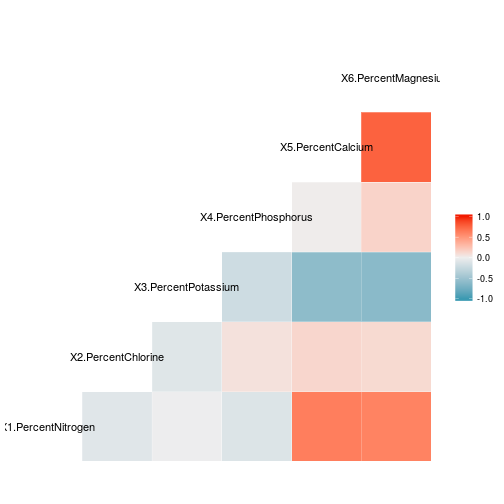GGally::ggcorr(tobacco_y)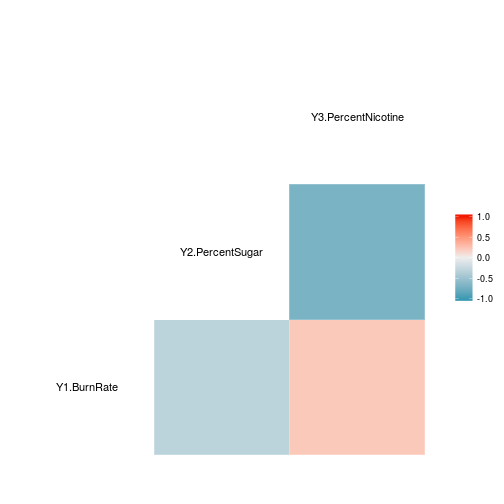## multivariate regression x <- as.matrix(tobacco_x) y <- as.matrix(tobacco_y) multivar_reg <- t(cov(y, x) %*% solve(cov(x))) ## separate multiple regression lm1 <- lm(y[,1] ~ x)$coeff
lm2 <- lm(y[,2] ~ x)$coeff lm3 <- lm(y[,3] ~ x)$coeff


As expected, the multivariate coefficients are the same as the multiple regression coefficients of each of the $Y_i$s

multivar_reg

##                      Y1.BurnRate Y2.PercentSugar Y3.PercentNicotine
## X1.PercentNitrogen    0.06197282      -4.3186662          0.5521620
## X2.PercentChlorine   -0.16012848       1.3262863         -0.2785609
## X3.PercentPotassium   0.29211810       1.5899470          0.2175877
## X4.PercentPhosphorus -0.65798016      13.9526510         -0.7231067
## X5.PercentCalcium     0.17302593       0.5525913          0.3230914
## X6.PercentMagnesium  -0.42834825      -3.5021083          2.0048603

cbind(lm1, lm2, lm3)

##                               lm1        lm2        lm3
## (Intercept)            1.41113730 13.6329133 -1.5648236
## xX1.PercentNitrogen    0.06197282 -4.3186662  0.5521620
## xX2.PercentChlorine   -0.16012848  1.3262863 -0.2785609
## xX3.PercentPotassium   0.29211810  1.5899470  0.2175877
## xX4.PercentPhosphorus -0.65798016 13.9526510 -0.7231067
## xX5.PercentCalcium     0.17302593  0.5525913  0.3230914
## xX6.PercentMagnesium  -0.42834825 -3.5021083  2.0048603


## Reduced-Rank Regression

One way to introduce a multivariate component into the model is to allow for the possibility that $$\mathbf{C}$$ is deficient, or of reduced-rank $$t$$.

$\mathrm{rank}\left(\mathbf{C}\right) = t \leq \mathrm{min}\left(r, s\right)$

In other words, we allow for the possibility that there are unknown linear constraints on $$\mathbf{C}$$.

Without loss of generality, we consider the case when $$r > s$$, i.e., $$t < s$$.

When $$t = s$$, the regression model is full-rank, and can be fit using multiple regression on each $$Y_i \in \mathbf{Y}.$$ When $$t < s$$, $$\mathbf{C}$$ can be decomposed into non-unique matrices $$\mathbf{A}_{s \times t}$$ and $$\mathbf{B}_{t \times r}$$, such that $$\mathbf{C} = \mathbf{AB},$$ and the multivariate regression model is given by

$\overset{s \times 1}{\mathbf{Y}} = \overset{s \times 1}{\boldsymbol{\mu}} + \overset{s \times t}{\mathbf{A}} \; \overset{t \times r}{\mathbf{B}} \; \overset{r \times 1}{\mathbf{X}} + \overset{s \times 1}{\varepsilon}$

Estimating $$\boldsymbol{\mu}, \mathbf{A}, \mathbf{B}$$, and ultimately the reduced-rank regression coefficient $$\mathbf{C}^{\left(t\right)}$$, is done by minimizing the weighted sum-of-squares criterion

$\mathrm{E}\left[\left(\mathbf{Y} - \boldsymbol{\mu} - \mathbf{ABX}\right)^\tau \mathbf{\Gamma}\left(\mathbf{Y} - \boldsymbol{\mu} - \mathbf{ABX}\right)\right]$

where $$\boldsymbol{\Gamma}$$ is a positive-definite symmetric $$\left(s \times s\right)$$-matrix of weights, the expectation of which is taken over the joint distribution $$\left(\mathbf{X}^\tau, \mathbf{Y}^\tau\right)^\tau$$. This weighted sum-of-squares criterion is minimized when

\begin{aligned} \boldsymbol{\mu}^{\left(t\right)} & = \boldsymbol{\mu}_Y - \mathbf{A}^{\left(t\right)}\mathbf{B}^{\left(t\right)}\boldsymbol{\mu}_X \ \mathbf{A}^{\left(t\right)} & = \mathbf{\Gamma}^{-½}\mathbf{V}_t \ \mathbf{B}^{\left(t\right)} & = \mathbf{V}_t^\tau \boldsymbol{\Gamma}^{-½}\mathbf{\Sigma}_{YX}\mathbf{\Sigma}_{XX}^{-1} \ \end{aligned}

where $$\mathbf{V}_t = \left(\mathbf{v}_1, \dots, \mathbf{v}_t\right)$$ is an $$\left(s \times t\right)$$-matrix, with $$\mathbf{v}_j$$ the eigenvector associated with the $j$th largest eigenvalue of

$\mathbf{\Gamma}^{½}\mathbf{\Sigma}_{YX} \mathbf{\Sigma}_{XX}^{-1} \mathbf{\Sigma}_{XY} \mathbf{\Gamma}^{½}$

In practice, we try out different values of $$\mathbf{\Gamma}$$. Two popular choices – and ones that lead to interesting results as we will see – are $$\mathbf{\Gamma} = \mathbf{I}_r$$ and $$\mathbf{\Gamma} = \boldsymbol{\Sigma}_{YY}^{-1}$$.

Since the reduced-rank regression coefficient relies on inverting $$\boldsymbol{\Sigma}_{XX}$$ and, possibly, $$\boldsymbol{\Sigma}_{YY}$$, we want to take into consideration the cases when $$\boldsymbol{\Sigma}_{XX}, \boldsymbol{\Sigma}_{YY}$$ are singular or difficult to invert.

Borrowing from ridge regression, we perturb the diagonal of the covariance matrices by some small constant, $$k$$. Thus, we carry out the reduced-rank regression procedure using

\begin{aligned} \hat{\boldsymbol{\Sigma}}_{XX}^{\left(k\right)} & = \hat{\boldsymbol{\Sigma}}_{XX} + k \mathbf{I}_r \ \hat{\boldsymbol{\Sigma}}_{YY}^{\left(k\right)} & = \hat{\boldsymbol{\Sigma}}_{YY} + k \mathbf{I}_r \end{aligned}

### Estimate $$t$$ and $$k$$ with rank_trace().

args(rank_trace)

## function (x, y, type = "identity", k = 0, plot = TRUE, interactive = FALSE)
## NULL


Since $$\hat{\mathbf{C}}$$ is calculated using sample observations, its mathematical rank will always be full, but it will have a statistical rank $$t$$ which is an unknown hyperparameter that needs to be estimated.

One method of estimating $$t$$ is to plot the rank trace. Along the $$X$$-axis, we plot a measure of the difference between the rank-$$t$$ coefficient matrix and the full-rank coefficient matrix for each value of $$t$$. Along the $$Y$$-axis, we plot the reduction in residual covariance between the rank-$$t$$ residuals and the full-rank residuals for each value of $$t$$.

### use the identity matrix for gamma

rank_trace(tobacco_x, tobacco_y)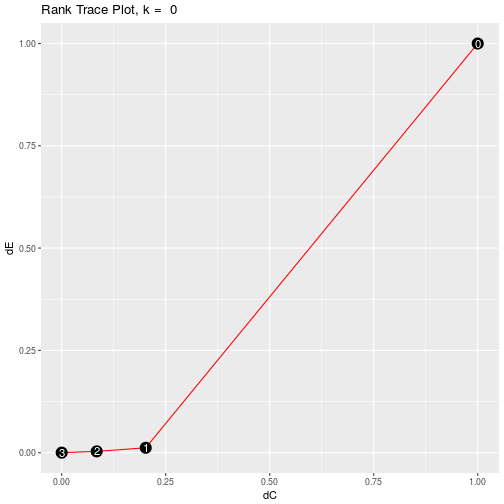Set plot = FALSE to print data frame of rank trace coordinates.

rank_trace(tobacco_x, tobacco_y, plot = FALSE)

## # A tibble: 4 × 3
##   ranks         dC         dEE
##   <int>      <dbl>       <dbl>
## 1     0 1.00000000 1.000000000
## 2     1 0.20198327 0.011933691
## 3     2 0.08419093 0.003095346
## 4     3 0.00000000 0.000000000


When the weight matrix, $$\mathbf{\Gamma}$$, takes on a more complicated form, the rank trace may plot points outside the unit square, or may not be a smooth monotic curve. When this is the case, we can change the value of k to smooth the rank trace. This value of $$\hat{k}$$ is then an estimate of the ridge pertubation $$k$$ described above.

The following rank trace is smooth, but we can always add a value $$k$$ to softly shrink[Aldrin, Magne. “Multivariate Prediction Using Softly Shrunk Reduced-Rank Regression.” The American Statistician 54.1 (2000): 29. Web. ] the reduced-rank regression.

### use inverse of estimated covariance of Y for gamma

rank_trace(tobacco_x, tobacco_y, type = "cva")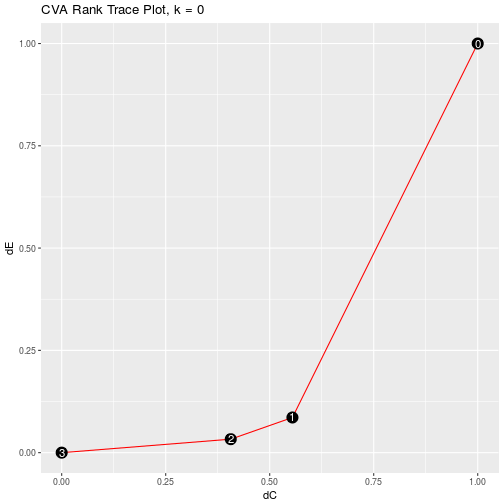#rank_trace(tobacco_x, tobacco_y, type = "cva", plot = FALSE)


### Fitting Reduced-Rank Regression Model

The main function in the rrr package is – unsurprisingly – rrr() which fits a reduced-rank regression model and outputs the coefficients.

### Fit reduced-rank regression model with rrr()

args(rrr)

## function (x, y, type = "identity", rank = "full", k = 0)
## NULL


rrr() takes as inputs the data frames, or matrices, of input and response variables, the weight matrix $$\mathbf{\Gamma}$$, the rank (defaulted to full rank), the type of covariance matrix to be used (either covariance or correlation), and the ridge constant $$k$$.

rrr() returns a list containing the means $$\hat{\boldsymbol{\mu}}$$, the matrices $$\hat{\mathbf{A}}$$, $$\hat{\mathbf{B}}$$, and the coefficient matrix $$\hat{\mathbf{C}}$$, as well as the eigenvalues of the weight marix $$\mathbf{\Gamma}$$.

rrr(tobacco_x, tobacco_y, rank = "full")

## $mean ## [,1] ## Y1.BurnRate 1.411137 ## Y2.PercentSugar 13.632913 ## Y3.PercentNicotine -1.564824 ## ##$A
##                           [,1]       [,2]      [,3]
## Y1.BurnRate         0.03107787 -0.4704307 0.8818895
## Y2.PercentSugar    -0.97005030  0.1984637 0.1400521
## Y3.PercentNicotine  0.24090782  0.8598297 0.4501736
##
## $B ## X1.PercentNitrogen X2.PercentChlorine X3.PercentPotassium ## [1,] 4.3242696 -1.35864835 -1.4808316 ## [2,] -0.4114869 0.09903401 0.3652138 ## [3,] -0.3016163 -0.08086722 0.5782436 ## X4.PercentPhosphorus X5.PercentCalcium X6.PercentMagnesium ## [1,] -13.729424 -0.4528289 3.86689562 ## [2,] 2.456879 0.3060762 1.23030547 ## [3,] 1.048309 0.3754285 0.03430168 ## ##$C
##                    X1.PercentNitrogen X2.PercentChlorine
## Y1.BurnRate                0.06197282         -0.1601285
## Y2.PercentSugar           -4.31866620          1.3262863
## Y3.PercentNicotine         0.55216201         -0.2785609
##                    X3.PercentPotassium X4.PercentPhosphorus
## Y1.BurnRate                  0.2921181           -0.6579802
## Y2.PercentSugar              1.5899470           13.9526510
## Y3.PercentNicotine           0.2175877           -0.7231067
##                    X5.PercentCalcium X6.PercentMagnesium
## Y1.BurnRate                0.1730259          -0.4283482
## Y2.PercentSugar            0.5525913          -3.5021083
## Y3.PercentNicotine         0.3230914           2.0048603
##
## eigen_values ##  3.28209974 0.03782978 0.01015996  We can see that rrr() with rank = "full" and k = 0 returns the classical multivariate regression coefficients as above. They differ only by a transpose, and is presented this way in rrr as a matter of convention. It is this form that is presented in the literature.[Izenman, A.J. (2008) Modern Multivariate Statistical Techniques. Springer. ] ### Diagnostics ### Calculate Residuals with residuals() args(residuals)  ## function (x, y, type = "identity", rank = "full", k = 0, plot = TRUE) ## NULL  residuals(tobacco_x, tobacco_y, rank = 1, plot = FALSE)  ## # A tibble: 25 × 3 ## Y1.BurnRate Y2.PercentSugar Y3.PercentNicotine ## <dbl> <dbl> <dbl> ## 1 -0.15358056 3.9207245 -0.9019766 ## 2 -0.20963638 0.6975085 -0.6966471 ## 3 -0.07296342 3.3478677 -0.9497452 ## 4 -0.20128009 2.9831896 -0.1991789 ## 5 -0.12358413 1.6364610 -0.3622142 ## 6 -0.04949370 0.3195653 -1.2428487 ## 7 -0.04185307 2.0824285 -0.3387954 ## 8 -0.14497977 2.7465342 -0.1903404 ## 9 -0.09788965 1.5330911 -0.5878621 ## 10 -0.35531326 2.6111104 -0.3332054 ## # ... with 15 more rows  ### Plot Residuals We can visually check the model assumptions with residuals(). The leftmost column of the scatter plot can be used to look for serial patterns in the residuals. The diagonal can be used to look at the distribution and visually assess whether or not it is symmetric, has a mean of zero, etc. residuals(tobacco_x, tobacco_y, rank = 1)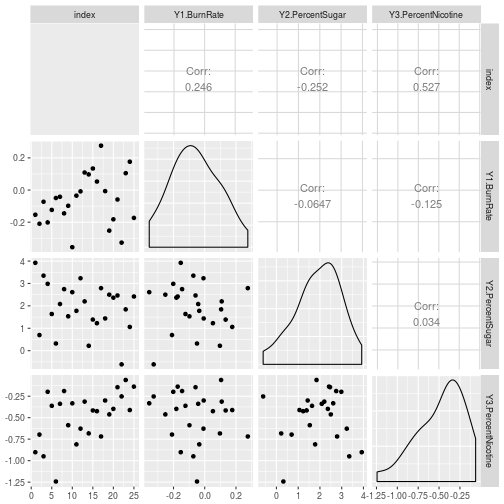To print a data frame of the residuals, set plot = FALSE. residuals(tobacco_x, tobacco_y, rank = 1, plot = FALSE)  ## # A tibble: 25 × 3 ## Y1.BurnRate Y2.PercentSugar Y3.PercentNicotine ## <dbl> <dbl> <dbl> ## 1 -0.15358056 3.9207245 -0.9019766 ## 2 -0.20963638 0.6975085 -0.6966471 ## 3 -0.07296342 3.3478677 -0.9497452 ## 4 -0.20128009 2.9831896 -0.1991789 ## 5 -0.12358413 1.6364610 -0.3622142 ## 6 -0.04949370 0.3195653 -1.2428487 ## 7 -0.04185307 2.0824285 -0.3387954 ## 8 -0.14497977 2.7465342 -0.1903404 ## 9 -0.09788965 1.5330911 -0.5878621 ## 10 -0.35531326 2.6111104 -0.3332054 ## # ... with 15 more rows  ## Principal Components Analysis ### PCA is a Special Case of Reduced-Rank Regression Set \begin{aligned} \mathbf{Y} & \equiv \mathbf{X} \ \mathbf{\Gamma} & = \mathbf{I}_r \end{aligned} Then, the least squares criterion $\mathrm{E}\left[\left(\mathbf{X} - \boldsymbol{\mu} - \mathbf{A}\mathbf{B} \mathbf{X}\right)\left(\mathbf{X} - \boldsymbol{\mu} - \mathbf{A}\mathbf{B} \mathbf{X}\right)^\tau\right]$ is minimized when \begin{aligned} \mathbf{A}^{\left(t\right)} & = \left(\mathbf{v}_1, \dots, \mathbf{v}_t\right) \ \mathbf{B}^{\left(t\right)} & = \mathbf{A}^{\left(t\right) \tau} \ \boldsymbol{\mu}^{\left(t\right)} & = \left(\mathbf{I}_r - \mathbf{A}^{\left(t\right)}\mathbf{B}^{\left(t\right)}\right)\boldsymbol{\mu}_X \ \end{aligned} where $$\mathbf{v}_j = \mathbf{v}_j \left(\mathbf{\Sigma}_{XX}\right)$$ is the eigenvector associated with thejth largest eigenvalue of $$\mathbf{\Sigma}_{XX}.$$ The best reduced-rank approximation to the original $$\mathbf{X}$$ is \begin{aligned} \hat{\mathbf{X}}^{\left(t\right)} & = \boldsymbol{\mu}^{\left(t\right)} + \mathbf{A}^{\left(t\right)}\mathbf{B}^{\left(t\right)} \mathbf{X} \ & \mathrm{or} \ \hat{\mathbf{X}} & = \mathbf{A}^{\left(t\right)}\mathbf{B}^{\left(t\right)}\mathbf{X}_c \ \end{aligned} where $$\mathbf{X}_c$$ is the vector $$\mathbf{X}$$ after mean-centering. The first principle component is a latent variable that is a linear combination of theX_i$s that maximizes the variance among the$X_i$s. The second principle component is another linear combination that maximizes the variance among the$X_i$s subject to being independent of the first principal component. There are $$r$$ possible principal components, each independent of each other, that capture decreasing amounts of variance. The goal is to use as few principle components as necessary to capture the variance in the data and reduce dimensionality. The question of how many principle components to keep is equivalent to assessing the effective dimensionality $$t$$ of the reduced-rank regression. ## The pendigits Data Set data(pendigits) digits <- as_data_frame(pendigits) %>% select(-V36) glimpse(digits)  ## Observations: 10,992 ## Variables: 35 ##$ V1  <int> 47, 0, 0, 0, 0, 100, 0, 0, 13, 57, 74, 48, 100, 91, 0, 35,...
## $V2 <int> 100, 89, 57, 100, 67, 100, 100, 39, 89, 100, 87, 96, 100, ... ##$ V3  <int> 27, 27, 31, 7, 49, 88, 3, 2, 12, 22, 31, 62, 72, 54, 38, 5...
## $V4 <int> 81, 100, 68, 92, 83, 99, 72, 62, 50, 72, 100, 65, 99, 100,... ##$ V5  <int> 57, 42, 72, 5, 100, 49, 26, 11, 72, 0, 0, 88, 36, 0, 81, 1...
## $V6 <int> 37, 75, 90, 68, 100, 74, 35, 5, 38, 31, 69, 27, 78, 87, 88... ##$ V7  <int> 26, 29, 100, 19, 81, 17, 85, 63, 56, 25, 62, 21, 34, 23, 8...
## $V8 <int> 0, 45, 100, 45, 80, 47, 35, 0, 0, 0, 64, 0, 54, 59, 50, 66... ##$ V9  <int> 0, 15, 76, 86, 60, 0, 100, 100, 4, 75, 100, 21, 79, 81, 84...
## $V10 <int> 23, 15, 75, 34, 60, 16, 71, 43, 17, 13, 79, 33, 47, 67, 12... ##$ V11 <int> 56, 37, 50, 100, 40, 37, 73, 89, 0, 100, 100, 79, 64, 100,...
## $V12 <int> 53, 0, 51, 45, 40, 0, 97, 99, 61, 50, 38, 67, 13, 39, 0, 9... ##$ V13 <int> 100, 69, 28, 74, 33, 73, 65, 36, 32, 75, 84, 100, 19, 79, ...
## $V14 <int> 90, 2, 25, 23, 20, 16, 49, 100, 94, 87, 0, 100, 0, 4, 22, ... ##$ V15 <int> 40, 100, 16, 67, 47, 20, 66, 0, 100, 26, 18, 0, 0, 21, 100...
## $V16 <int> 98, 6, 0, 0, 0, 20, 0, 57, 100, 85, 1, 85, 2, 0, 24, 17, 7... ##$ V17 <int> 8, 2, 1, 4, 1, 6, 4, 0, 5, 0, 9, 8, 5, 9, 7, 3, 3, 9, 2, 2...
## $V18 <int> 1, 2, 3, 6, 3, 4, 6, 10, 7, 5, 6, 1, 9, 6, 2, 9, 9, 9, 2, ... ##$ V19 <int> 47, 0, 0, 0, 0, 100, 0, 0, 13, 57, 74, 48, 100, 91, 0, 35,...
## $V20 <int> 100, 89, 57, 100, 67, 100, 100, 39, 89, 100, 87, 96, 100, ... ##$ V21 <int> 27, 27, 31, 7, 49, 88, 3, 2, 12, 22, 31, 62, 72, 54, 38, 5...
## $V22 <int> 81, 100, 68, 92, 83, 99, 72, 62, 50, 72, 100, 65, 99, 100,... ##$ V23 <int> 57, 42, 72, 5, 100, 49, 26, 11, 72, 0, 0, 88, 36, 0, 81, 1...
## $V24 <int> 37, 75, 90, 68, 100, 74, 35, 5, 38, 31, 69, 27, 78, 87, 88... ##$ V25 <int> 26, 29, 100, 19, 81, 17, 85, 63, 56, 25, 62, 21, 34, 23, 8...
## $V26 <int> 0, 45, 100, 45, 80, 47, 35, 0, 0, 0, 64, 0, 54, 59, 50, 66... ##$ V27 <int> 0, 15, 76, 86, 60, 0, 100, 100, 4, 75, 100, 21, 79, 81, 84...
## $V28 <int> 23, 15, 75, 34, 60, 16, 71, 43, 17, 13, 79, 33, 47, 67, 12... ##$ V29 <int> 56, 37, 50, 100, 40, 37, 73, 89, 0, 100, 100, 79, 64, 100,...
## $V30 <int> 53, 0, 51, 45, 40, 0, 97, 99, 61, 50, 38, 67, 13, 39, 0, 9... ##$ V31 <int> 100, 69, 28, 74, 33, 73, 65, 36, 32, 75, 84, 100, 19, 79, ...
## $V32 <int> 90, 2, 25, 23, 20, 16, 49, 100, 94, 87, 0, 100, 0, 4, 22, ... ##$ V33 <int> 40, 100, 16, 67, 47, 20, 66, 0, 100, 26, 18, 0, 0, 21, 100...
## $V34 <int> 98, 6, 0, 0, 0, 20, 0, 57, 100, 85, 1, 85, 2, 0, 24, 17, 7... ##$ V35 <int> 8, 2, 1, 4, 1, 6, 4, 0, 5, 0, 9, 8, 5, 9, 7, 3, 3, 9, 2, 2...

digits_features <- digits %>% select(-V35)
digits_class <- digits %>% select(V35)


We can get a good visualization of the correlation structure using GGally::ggcorr. Below we see that there is very heavy correlation among the variables.

GGally::ggcorr(digits_features)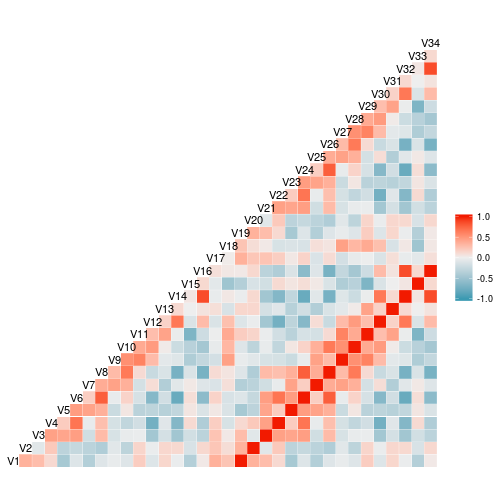### Assessing Dimensionality

The ratio

$\frac{\lambda_{t + 1} + \cdots \lambda_r}{\lambda_1 + \cdots \lambda_r}$

is a goodness-of-fit measure of how well the last $$r - t$$ principal components explain the totoal variation in $$\mathbf{X}$$

The function rrr() (see below) outputs this goodness-of-fit measure

rrr(digits_features, digits_features, type = "pca")$goodness_of_fit  ## PC1 PC2 PC3 PC4 PC5 ## 7.168777e-01 4.680599e-01 3.144671e-01 2.243293e-01 1.664009e-01 ## PC6 PC7 PC8 PC9 PC10 ## 1.181315e-01 8.737814e-02 6.065970e-02 4.138801e-02 2.763994e-02 ## PC11 PC12 PC13 PC14 PC15 ## 1.896530e-02 1.222037e-02 7.761240e-03 3.816942e-03 1.958674e-03 ## PC16 PC17 PC18 PC19 PC20 ## 3.133204e-04 1.279543e-04 -6.983068e-17 -8.770990e-17 -9.882538e-17 ## PC21 PC22 PC23 PC24 PC25 ## -1.092158e-16 -1.158156e-16 -1.172696e-16 -1.184188e-16 -1.145857e-16 ## PC26 PC27 PC28 PC29 PC30 ## -1.103970e-16 -1.053676e-16 -9.801713e-17 -8.865624e-17 -7.546966e-17 ## PC31 PC32 PC33 PC34 ## -6.161541e-17 -4.449590e-17 -2.257015e-17 0.000000e+00  ### Estimate $$t$$ and ridge constant $$k$$ with rank_trace() rank_trace(digits_features, digits_features, type = "pca")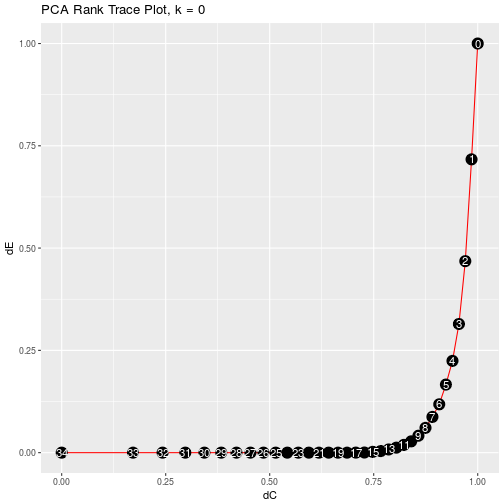Print data frame of rank trace coordinates by setting plot = FALSE. rank_trace(digits_features, digits_features, type = "pca", plot = FALSE)  ## # A tibble: 35 × 3 ## rank delta_C delta_residuals ## <int> <dbl> <dbl> ## 1 0 1.0000000 1.00000000 ## 2 1 0.9851844 0.71687775 ## 3 2 0.9701425 0.46805987 ## 4 3 0.9548637 0.31446713 ## 5 4 0.9393364 0.22432933 ## 6 5 0.9235481 0.16640087 ## 7 6 0.9074852 0.11813147 ## 8 7 0.8911328 0.08737814 ## 9 8 0.8744746 0.06065970 ## 10 9 0.8574929 0.04138801 ## # ... with 25 more rows  ### Plot Principal Component Scores ### Pairwise Plots with pairwise_plot() args(pairwise_plot)  ## function (x, y, type = "pca", pair_x = 1, pair_y = 2, rank = "full", ## k = 0, interactive = FALSE, point_size = 2.5) ## NULL  A common PCA method of visualization for diagnostic and analysis purposes is to plot the$j$th sample PC scores against the$kth PC scores, \begin{aligned} \left(\xi_{ij}, \xi_{ik}\right) & \ = \left(\hat{\mathbf{v}}_j^\tau \mathbf{X}_i, \hat{\mathbf{v}}_k^\tau \mathbf{X}_i\right)&, \quad i = 1,2, \dots, n \end{aligned} Since the first two principal components will capture the most variance – and hence the most useful information – of all possible pairs of principal components, we typically would set $$j = 1, k = 2$$ and plot the first two sample PC scores against each other. In rrr this is the default. pairwise_plot(digits_features, digits_class, type = "pca")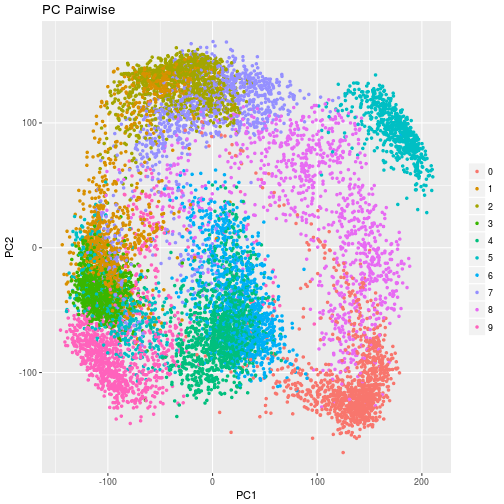We can set the $$x$$- and $$y$$-axes to whichever pairs of PC scores we would like to plot by changing the pc_x and pc_y arguments. pairwise_plot(digits_features, digits_class, type = "pca", pair_x = 1, pair_y = 3)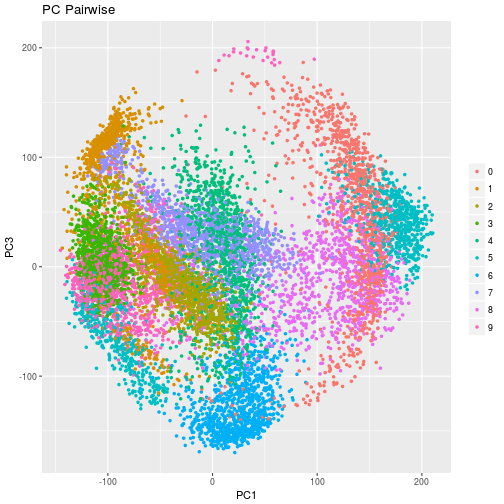### Plot all pairs of PC scores with pca_allpairs_plot() Alternatively, we can look at structure in the data by plotting all PC pairs, along with some other visual diagnostics with pca_allpairs_plot(). Along with plotting principal component scores against each other, the plot matrix also shows histograms and box plots to show how the points are distributed along principal component axes. #args(pca_allpairs_plot)  #pca_allpairs_plot(digits_features, rank = 3, class_labels = digits_class)  ### Fitting a PCA Model ### Fit model with rrr() rrr(digits_features, digits_features, type = "pca", rank = 3)  ##means
##        V1        V2        V3        V4        V5        V6        V7
## 38.814320 85.120269 40.605622 83.774199 49.770378 65.573144 51.220251
##        V8        V9       V10       V11       V12       V13       V14
## 44.498999 56.868541 33.695961 60.516376 34.826510 55.022289 34.937045
##       V15       V16       V17       V18       V19       V20       V21
## 47.287482 28.845342  4.431587  5.341794 38.814320 85.120269 40.605622
##       V22       V23       V24       V25       V26       V27       V28
## 83.774199 49.770378 65.573144 51.220251 44.498999 56.868541 33.695961
##       V29       V30       V31       V32       V33       V34
## 60.516376 34.826510 55.022289 34.937045 47.287482 28.845342
##
## $C ## # A tibble: 34 × 34 ## V1 V2 V3 V4 V5 ## <dbl> <dbl> <dbl> <dbl> <dbl> ## 1 0.15227028 0.041971586 0.057621563 0.032824603 -0.083070856 ## 2 0.04197159 0.013118640 0.010598715 0.005435764 -0.027861499 ## 3 0.05762156 0.010598715 0.057059768 0.042972135 0.005136858 ## 4 0.03282460 0.005435764 0.042972135 0.034784478 0.014445469 ## 5 -0.08307086 -0.027861499 0.005136858 0.014445469 0.083611542 ## 6 -0.00509760 -0.010118470 0.048612391 0.041246125 0.054433598 ## 7 -0.12925579 -0.039313730 -0.047145641 -0.030698476 0.070011543 ## 8 -0.03613352 -0.021185046 0.025475341 0.019298193 0.056664934 ## 9 -0.04375152 -0.018001465 -0.036555354 -0.038171115 -0.002983700 ## 10 -0.05163631 -0.020586646 -0.022911782 -0.022807068 0.019987392 ## # ... with 24 more rows, and 29 more variables: V6 <dbl>, V7 <dbl>, ## # V8 <dbl>, V9 <dbl>, V10 <dbl>, V11 <dbl>, V12 <dbl>, V13 <dbl>, ## # V14 <dbl>, V15 <dbl>, V16 <dbl>, V17 <dbl>, V18 <dbl>, V19 <dbl>, ## # V20 <dbl>, V21 <dbl>, V22 <dbl>, V23 <dbl>, V24 <dbl>, V25 <dbl>, ## # V26 <dbl>, V27 <dbl>, V28 <dbl>, V29 <dbl>, V30 <dbl>, V31 <dbl>, ## # V32 <dbl>, V33 <dbl>, V34 <dbl> ## ##$PC
## # A tibble: 34 × 3
##            PC1          PC2         PC3
##          <dbl>        <dbl>       <dbl>
## 1   0.02861158 -0.142768395 -0.36203431
## 2   0.04680878 -0.033512929 -0.09901748
## 3  -0.13901701  0.046687918 -0.18855842
## 4  -0.10267046  0.083217740 -0.13159811
## 5  -0.15980723  0.196663538  0.13927183
## 6  -0.24050660  0.117714673 -0.05134764
## 7  -0.10613246  0.031714447  0.33613210
## 8  -0.28960021 -0.002314316  0.07783244
## 9  -0.11746249 -0.263105908  0.21532196
## 10 -0.14441246 -0.150734547  0.19065755
## # ... with 24 more rows
##
## $goodness_of_fit ## PC1 PC2 PC3 ## 0.7168777 0.4680599 0.3144671  ## Canonical Variate Analysis ### CVA as a Special Case of Reduced-Rank Regression Canonical Variate Analysis[Hotelling, H. (1936). Relations between two sets of variates, Biometrika, 28, 321-377.] is a method of linear dimensionality reduction. It is assumed that $$\left(\mathbf{X}, \mathbf{Y}\right)$$ are jointly distributed with $\mathrm{E}\left\{ \begin{pmatrix} \mathbf{X}\ \mathbf{Y}\ \end{pmatrix} \right\} = \begin{pmatrix} \boldsymbol{\mu}_X \ \bodlsybmol{\mu}_Y \ \end{pmatrix}, \quad \mathrm{cov}\left\{ \begin{pmatrix} \mathbf{X}\ \mathbf{Y}\ \end{pmatrix} \right\} = \begin{pmatrix} \mathbf{\Sigma}_{XX} & \mathbf{\Sigma}_{XY} \ \mathbf{\Sigma}_{YX} & \mathbf{\Sigma}_{YY} \ \end{pmatrix}$ The $$t$$ new pairs of canonical variables $$\left(\xi_i, \omega_i\right), i = 1, \dots, t$$ are calculated by fitting a reduced rank regression equation. The canonical variate scores are given by $\mathbf{\xi}^{\left(t\right)} = \mathbf{G}^{\left(t\right)}\mathbf{X}, \quad \mathbf{\omega}^{\left(t\right)} = \mathbf{H}^{\left(t\right)} \mathbf{Y},$ with $\mathbf{\Gamma} & = \mathbf{\Sigma}_{YY}^{-1} \ \mathbf{G}^{\left(t\right)} & = \mathbf{B}^{\left(t\right)} \ \mathbf{H}^{\left(t\right)} & = \mathbf{A}^{\left(t\right)-} \$ where $$\mathbf{A}^{\left(t\right)}, \mathbf{B}^{\left(t\right)}$$ are the matrices from the reduced-rank regression formulation above. Note that $$\mathbf{H}^{\left(t\right)} = \mathbf{A}^{\left(t\right)-}$$ is the generalized inverse of $$\mathbf{A}^{\left(t\right)}$$. When $$t = s, \mathbf{H}^{\left(s\right)} = \mathbf{A}^{\left(t\right)+}$$ is the unique Moore-Penrose generalized inverse of $$\mathbf{A}^{\left(t\right)}$$. ## The COMBO17 Data Set ### COMBO-17 galaxy data data(COMBO17) galaxy <- as_data_frame(COMBO17) %>% select(-starts_with("e."), -Nr, -UFS:-IFD) %>% na.omit() glimpse(galaxy)  ## Observations: 3,462 ## Variables: 29 ##$ Rmag    <dbl> 24.995, 25.013, 24.246, 25.203, 25.504, 23.740, 25.706...
## $ApDRmag <dbl> 0.935, -0.135, 0.821, 0.639, -1.588, -1.636, 0.199, -0... ##$ mumax   <dbl> 24.214, 25.303, 23.511, 24.948, 24.934, 24.609, 25.271...
## $Mcz <dbl> 0.832, 0.927, 1.202, 0.912, 0.848, 0.882, 0.896, 0.930... ##$ MCzml   <dbl> 1.400, 0.864, 1.217, 0.776, 1.330, 0.877, 0.870, 0.877...
## $chi2red <dbl> 0.64, 0.41, 0.92, 0.39, 1.45, 0.52, 1.31, 1.84, 1.03, ... ##$ UjMAG   <dbl> -17.67, -18.28, -19.75, -17.83, -17.69, -19.22, -17.09...
## $BjMAG <dbl> -17.54, 17.86, -19.91, -17.39, -18.40, -18.11, -16.06,... ##$ VjMAG   <dbl> -17.76, -18.20, -20.41, -17.67, -19.37, -18.70, -16.23...
## $usMAG <dbl> -17.83, -18.42, -19.87, -17.98, -17.81, -19.34, -17.26... ##$ gsMAG   <dbl> -17.60, -17.96, -20.05, -17.47, -18.69, -18.27, -16.11...
## $rsMAG <dbl> -17.97, -18.43, -20.71, -17.89, -19.88, -19.05, -16.39... ##$ UbMAG   <dbl> -17.76, -18.36, -19.82, -17.92, -17.76, -19.30, -17.19...
## $BbMAG <dbl> -17.53, -17.85, -19.89, -17.38, -18.35, -18.08, -16.05... ##$ VnMAG   <dbl> -17.76, -18.19, -20.40, -17.67, -19.37, -18.69, -16.22...
## $S280MAG <dbl> -1.822e+01, -1.797e+01, -1.977e+01, -1.812e+01, -1.393... ##$ W420FE  <dbl> 0.0006600, 0.0003240, 0.0129700, 0.0118600, 0.0013450,...
## $W462FE <dbl> 0.012700, 0.005135, 0.019670, 0.015900, 0.005088, 0.00... ##$ W485FD  <dbl> 0.0188500, 0.0027290, 0.0255200, 0.0015550, 0.0018450,...
## $W518FE <dbl> 0.0182300, 0.0007852, 0.0159200, 0.0026140, 0.0099620,... ##$ W571FS  <dbl> 0.014680, 0.009910, 0.022890, 0.001756, 0.003439, 0.00...
## $W604FE <dbl> 0.016640, 0.009047, 0.023380, 0.009163, 0.006316, 0.00... ##$ W646FD  <dbl> 0.0188000, 0.0029790, 0.0231200, 0.0063330, -0.0001841...
## $W696FE <dbl> 0.024620, 0.009830, 0.027220, 0.012330, 0.005536, 0.00... ##$ W753FE  <dbl> 0.0244700, 0.0142100, 0.0354400, 0.0022500, 0.0161700,...
## $W815FS <dbl> 0.021560, 0.014710, 0.045340, 0.016880, 0.006755, 0.00... ##$ W856FD  <dbl> 0.024410, 0.011420, 0.078100, 0.008749, 0.010220, 0.00...
## $W914FD <dbl> 0.0377200, 0.0102800, 0.0711400, 0.0069970, 0.0132800,... ##$ W914FE  <dbl> 0.011660, 0.026270, 0.064050, 0.005865, 0.019850, 0.02...

galaxy_x <- galaxy %>%
select(-Rmag:-chi2red)

galaxy_y <- galaxy %>%
select(Rmag:chi2red)

GGally::ggcorr(galaxy_x)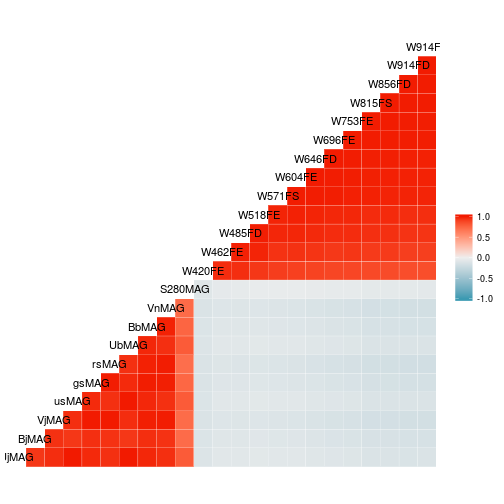GGally::ggcorr(galaxy_y)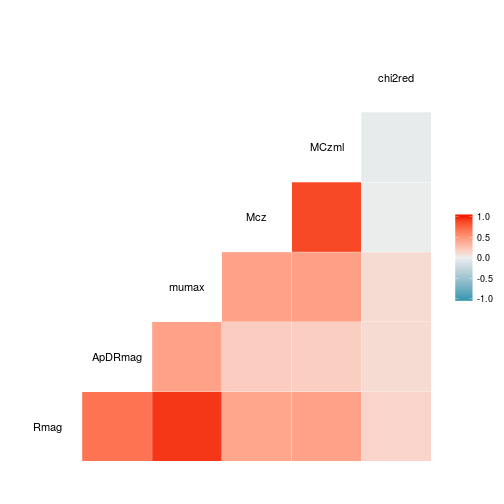### Assessing Effective Dimensionality

Estimate $$t$$ and $$k$$ with rank_trace()

rank_trace(galaxy_x, galaxy_y, type = "cva")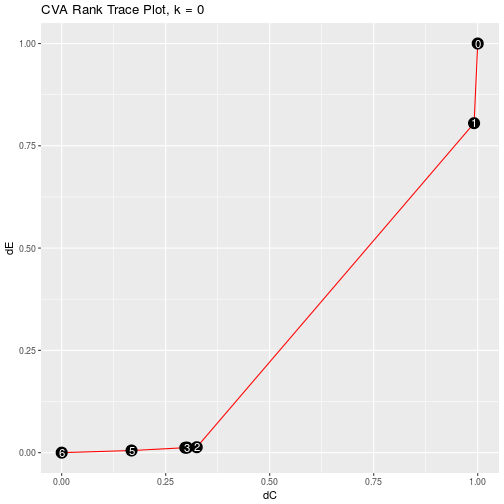### Diagnostics

Calculate residuals with residuals(), setting type = "cva".

residuals(galaxy_x, galaxy_y, type = "cva", rank = 2, k = 0.001, plot = FALSE)

## # A tibble: 3,462 × 3
##    index        CV1        CV2
##    <int>      <dbl>      <dbl>
## 1      1 -0.3202596  0.5609487
## 2      2 -0.3373066 -0.3235156
## 3      3  0.2342563  0.2243338
## 4      4  0.2188205  0.6709930
## 5      5 -0.7183237  1.5344605
## 6      6 -1.0225019  0.1872628
## 7      7  0.2784014  0.5923622
## 8      8 -0.1242796  0.6893633
## 9      9  0.2544044  0.2704908
## 10    10 -0.6856947 -0.1686793
## # ... with 3,452 more rows


### Plot Residuals

Plot residuals with residuals, setting type = "cva"

residuals(galaxy_x, galaxy_y, type = "cva", rank = 2, k = 0.001)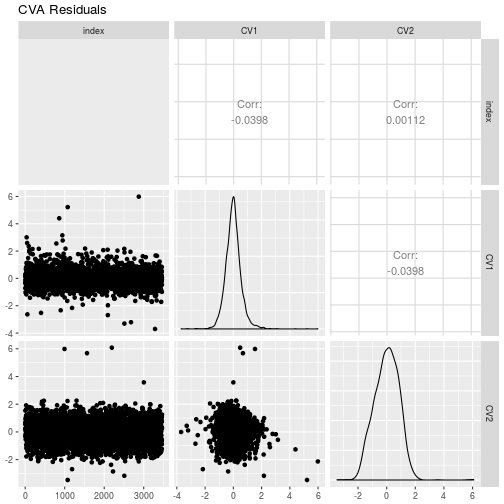### Plot Pairwise Canonical Variate Scores with pairwise_plot()

pairwise_plot(galaxy_x, galaxy_y, type = "cva", pair_x = 1, k = 0.0001)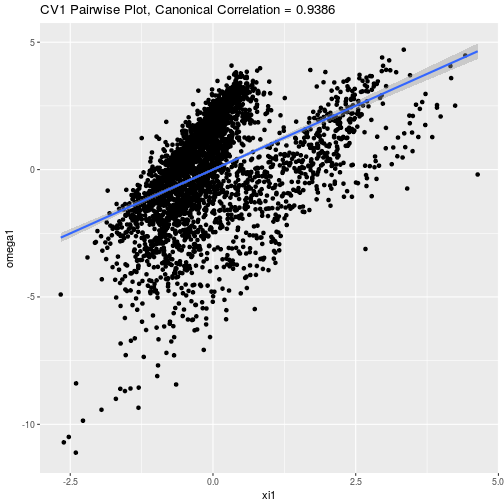pairwise_plot(galaxy_x, galaxy_y, type = "cva", pair_x = 2, k = 0.0001)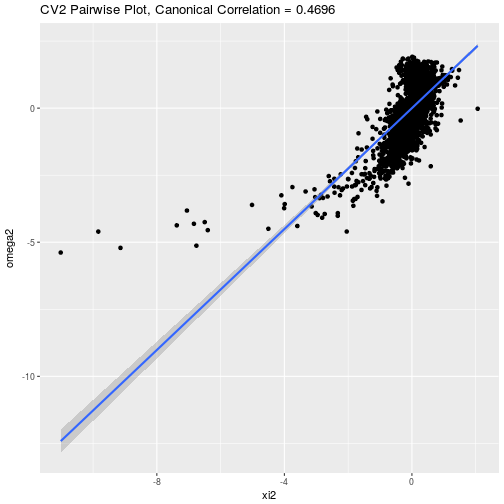pairwise_plot(galaxy_x, galaxy_y, type = "cva", pair_x = 3)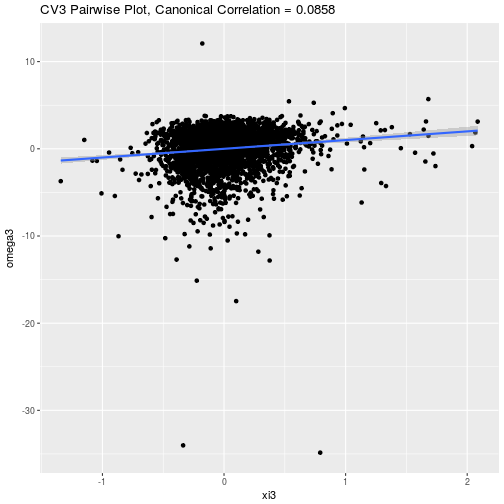pairwise_plot(galaxy_x, galaxy_y, type = "cva", pair_x = 6)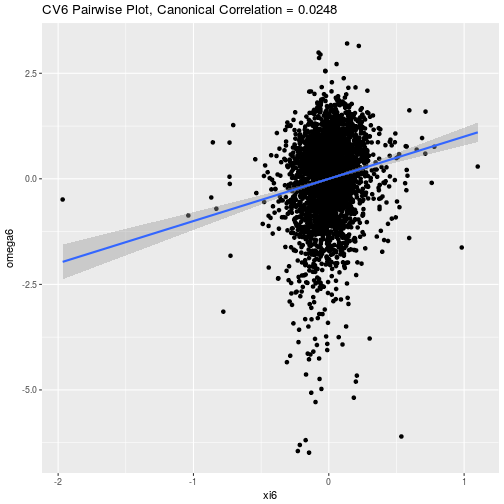### Fit Reduced-Rank Canonical Variate Model

Fit model with rrr(), setting type = "cva".

rrr(galaxy_x, galaxy_y, type = "cva", rank = 2, k = 0.0001)

## $mean ## [,1] ## Rmag 28.388670 ## ApDRmag 1.087370 ## mumax 26.721374 ## Mcz -1.166768 ## MCzml -1.025344 ## chi2red 2.080132 ## ##$G
##            UjMAG       BjMAG      VjMAG      usMAG       gsMAG      rsMAG
## [1,]  0.08245937 0.002984345  0.8404642 0.06621581 -0.08236586 -0.5801623
## [2,] -1.49675657 0.025440724 -3.5397513 2.13792464  0.85714917  2.9283156
##           UbMAG       BbMAG       VnMAG     S280MAG     W420FE    W462FE
## [1,]  0.2621465 -0.13452477  0.01348103 0.005561194  -1.486574 -1.270471
## [2,] -1.0059161  0.04282791 -0.05596699 0.036077743 -15.768191 -6.405781
##         W485FD     W518FE     W571FS    W604FE     W646FD     W696FE
## [1,] -1.241862 -0.0135883 -0.4363435 0.6564723  0.9527079  0.7664007
## [2,] -1.115042  8.1665877  3.6251209 3.6098337 -1.4617020 -5.0376775
##        W753FE     W815FS      W856FD    W914FD     W914FE
## [1,] 1.769078  0.1174032 -0.09706001 -1.957314 -0.4242234
## [2,] 3.334887 -8.2879583 -0.73423587  2.118483  1.9730962
##
## $H ## Rmag ApDRmag mumax Mcz MCzml chi2red ## [1,] 0.5617072 0.4771916 -0.1235694 -1.4243677 -1.3820735 0.5999989 ## [2,] 0.2899655 -0.1054661 0.4309957 0.5848668 0.5736387 -0.2302836 ## ##$canonical_corr
##  0.93863397 0.46957833 0.07553122 0.02573175 0.01503215 0.01113977


## Linear Discriminant Analysis

### LDA as a Special Case of CVA

Linear discriminant analysis is a classification procedure. We can turn it into a regression procedure – specifically a reduced-rank canonical variate procedure – in the following way.

Let each $$i = 1, 2, \dots, n$$ observation belong to one, and only one, of $$K = s + 1$$ distinct classes.

We can construct an indicator response matrix, $$\mathbf{Y}$$ where each row $$i$$ is an indicator response vector for the $i$th observation. The vector will have a 1 in the column that represents that class to which the observation belongs and will be 0 elsewhere.

We then regress this $$Y$$ binary response matrix against the matrix $$X$$ of predictor variables.

Linear discriminant analysis requires the assumptions that each class is normally distributed and that the covariance matrix of each class is equal to all others.

While these assumptions will not be met in all cases, when they are – and when the classes are well separated – linear discriminant analysis is a very efficient classification method.

## The iris Data Set

data(iris)
iris <- as_data_frame(iris)

glimpse(iris)

## Observations: 150
## Variables: 5
## $Sepal.Length <dbl> 5.1, 4.9, 4.7, 4.6, 5.0, 5.4, 4.6, 5.0, 4.4, 4.9,... ##$ Sepal.Width  <dbl> 3.5, 3.0, 3.2, 3.1, 3.6, 3.9, 3.4, 3.4, 2.9, 3.1,...
## $Petal.Length <dbl> 1.4, 1.4, 1.3, 1.5, 1.4, 1.7, 1.4, 1.5, 1.4, 1.5,... ##$ Petal.Width  <dbl> 0.2, 0.2, 0.2, 0.2, 0.2, 0.4, 0.3, 0.2, 0.2, 0.1,...
## $Species <fctr> setosa, setosa, setosa, setosa, setosa, setosa, ...  iris_features <- iris %>% select(-Species) iris_class <- iris %>% select(Species)  ### Assesssing Effective Dimensionality Assessing the rank $$t$$ of this reduced-rank regression is equivalent to determining the number of linear discriminant functions that best discriminate between the $$K$$ classes, with $$\mathrm{min}\left(r, s\right) = \mathrm{min}\left(r, K - 1\right)$$ maximum number of linear discriminant functions. Generally, plotting linear discriminant functions against each other, i.e., the first and second linear discriminant functions, is used to determine whether sufficient discrimination is obtained. Plotting techniques are discussed in the following section. ### Plotting Plot LDA Pairs with pairwise_plot(), setting type = "pca". A typical graphical display for multiclass LDA is to plot the$j$th discriminant scores for the $$n$$ points against the $$k$$ discriminant scores. pairwise_plot(iris_features, iris_class, type = "lda", k = 0.0001)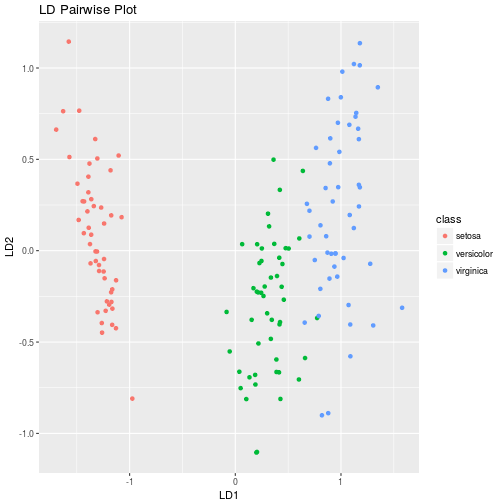### Fitting LDA Models Fit LDA model with rrr(), setting type = "lda". rrr(iris_features, iris_class, type = "lda", k = 0.0001)  ##$G
## # A tibble: 4 × 2
##          LD1          LD2
##        <dbl>        <dbl>
## 1 -0.1427550  0.009711862
## 2 -0.2639139  0.905610891
## 3  0.3792278 -0.388369442
## 4  0.4826420  1.184645528
##
## $H ## # A tibble: 2 × 2 ## LD1 LD2 ## <dbl> <dbl> ## 1 -1.123703 -0.3319998 ## 2 -0.265336 -1.1058423  ### Calculate LDA Scores with scores() scores(iris_features, iris_class, type = "lda", k = 0.0001)  ##$scores
## # A tibble: 150 × 3
##          LD1          LD2  class
##        <dbl>        <dbl> <fctr>
## 1  -1.387251  0.125583983 setosa
## 2  -1.226743 -0.329163835 setosa
## 3  -1.288897 -0.111147085 setosa
## 4  -1.172385 -0.280353248 setosa
## 5  -1.399367  0.215173886 setosa
## 6  -1.325346  0.611160171 setosa
## 7  -1.241218  0.148631516 setosa
## 8  -1.308661 -0.004785237 setosa
## 9  -1.128974 -0.424580855 setosa
## 10 -1.263476 -0.395904243 setosa
## # ... with 140 more rows
##
## \$class_means
## # A tibble: 3 × 3
##          LD1        LD2      class
##        <dbl>      <dbl>     <fctr>
## 1 -1.3498742  0.4053488     setosa
## 2  0.3239739 -1.3716811 versicolor
## 3  1.0259003  0.9663322  virginica